Test Description

## 20 Questions MCQ Test Mathematics (Maths) Class 8 | Olympiad Test: Mensuration

Olympiad Test: Mensuration for Class 8 2022 is part of Mathematics (Maths) Class 8 preparation. The Olympiad Test: Mensuration questions and answers have been prepared according to the Class 8 exam syllabus.The Olympiad Test: Mensuration MCQs are made for Class 8 2022 Exam. Find important definitions, questions, notes, meanings, examples, exercises, MCQs and online tests for Olympiad Test: Mensuration below.
Solutions of Olympiad Test: Mensuration questions in English are available as part of our Mathematics (Maths) Class 8 for Class 8 & Olympiad Test: Mensuration solutions in Hindi for Mathematics (Maths) Class 8 course. Download more important topics, notes, lectures and mock test series for Class 8 Exam by signing up for free. Attempt Olympiad Test: Mensuration | 20 questions in 20 minutes | Mock test for Class 8 preparation | Free important questions MCQ to study Mathematics (Maths) Class 8 for Class 8 Exam | Download free PDF with solutions
 1 Crore+ students have signed up on EduRev. Have you?
Olympiad Test: Mensuration - Question 1

### The parallel sides of a trapezium are 25 cm and 13 cm. Its non-parallel sides are equal, each being 10 cm. Find the area of the trapezium.

Detailed Solution for Olympiad Test: Mensuration - Question 1

Area of trapezium= 1/2*d*(a+b) sq. Units.

Where d= perpendicular distance between parellel sides which are a & b respectively, 25 &13 cm.

25–13=12, 12/2= 6 cm is base of RT. Angled ∆.

Non parallel sides=10cm, this is hypotenuese of this ∆.

Find ‘d ‘ to solve.

d=√{102–62}=8 cm.

Area of trapezium= 1/2*8*(25+13)=152 cm2

Olympiad Test: Mensuration - Question 2

### Find the area of the given quadrilateral.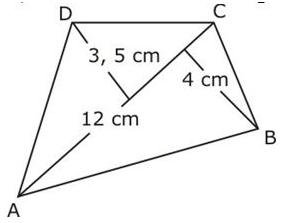Detailed Solution for Olympiad Test: Mensuration - Question 2

so, area(ABCD) = area(ABC) + area(ADC)

= 4 x 12/2 + 3.5 x12/2

= 24 + 21

= 45 cm2

Olympiad Test: Mensuration - Question 3

### Find the altitude of a trapezium, the sum of the lengths of whose bases is 6.5 cm and whose area is 26 cm2.

Olympiad Test: Mensuration - Question 4

Find the total surface area of a cube whose volume is 343 cm3.

Detailed Solution for Olympiad Test: Mensuration - Question 4
volume=(side)^3
so, 343=(side)^3
cube root of 343=side
cube root of 343=7
side=7cm
Surface area=6(side)^2
Surface area=6 x 7^2
Surface area=6 x 49
Surface area=294 cm^2
Olympiad Test: Mensuration - Question 5

A cylindrical tank has a capacity of 5632 m3. If the diameter of its base is 16 m, find its depth.

Olympiad Test: Mensuration - Question 6

Find the area of a rhombus whose diagonals are of lengths 20 cm and 16 cm.

Detailed Solution for Olympiad Test: Mensuration - Question 6

Area of rhombus =1/2 ×d1×d2
Length of the one diagonal= 20 cm
Length of the other diagonal =16 cm
Area of the rhombus =1/2×20×16
= 1×10×16
= 160
The area of the rhombus is 160cm^2.

Olympiad Test: Mensuration - Question 7

Find the area of the given figure.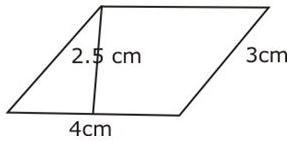Olympiad Test: Mensuration - Question 8

Surface area of a cuboid = __________

Detailed Solution for Olympiad Test: Mensuration - Question 8

Total Surface Area of Cuboid: 2(lb + bh + hl)
L = length
H = Height

Olympiad Test: Mensuration - Question 9

Find the volume of a cuboid whose length is 8 cm, breadth 6 cm and height 3.5 cm.

Detailed Solution for Olympiad Test: Mensuration - Question 9

Length of the cuboid = 8 cm

Breadth of the cuboid = 6 cm

Height of the cuboid = 3.5 cm

Volume of the cuboid = length × breadth × height

= 8 x 6 x 3.5 = 168cm3

Therefore,volume of  the cuboid = 168cm3

Olympiad Test: Mensuration - Question 10

A godown is in the form of a cuboid of measures 60 m × 40 m × 30 m. How many cuboidal boxes can be stored in it if the volume of one box is 0.8 m3?

Olympiad Test: Mensuration - Question 11

The perimeter of a trapezium is 52 cm. Its non-parallel sides are 10 cm each and the distance between two parallel sides is 8 cm. Find the area of the trapezium.

Detailed Solution for Olympiad Test: Mensuration - Question 11

Perimeter of a Trapezium=sum of all sides
52= sum of parallel sides +20
Sum of parallel sides = 32
Area of Trapezium=
1/2(Sum of parallel sides) x h
=1/2(32)*8 = 128cm2

Olympiad Test: Mensuration - Question 12

The cost of papering the wall of a room, 12 m long, at the rate of Rs. 1.35 per square meter is Rs. 340.20. The cost of matting the floor at Re. 0.85 per square metre is Rs. 91.80. Find the height of the room.

Detailed Solution for Olympiad Test: Mensuration - Question 12
Let the length of room = l = 12 m
breadth of room = b m
height of room = h m
Cost of matting the floor @ Re. 0.85 per m^2 = Rs. 91.80
floor area = 91.80 / 0.85 = 108 m^2
⇒ l * b = 108
⇒ 12 * b = 108
⇒ b = 108/12
⇒ b = 9 m
cost of papering wall @ Rs. 1.35 per m^2 = Rs. 340.20
wall area is the lateral surface area.
wall area = lateral surface area = 340.20 / 1.35 = 252 m^2
⇒ 2h(l+b) = 252
⇒ 2h(12+9) = 252
⇒ 2h*21 = 252
⇒ h = 252/(2*21)
⇒ h = 6 m
Height of room is 6m.
Olympiad Test: Mensuration - Question 13

The area of a trapezium is 384 cm2. Its parallel sides are in the ratio 3:5 and the distance between them is 12 cm. Find the length of each parallel side.

Olympiad Test: Mensuration - Question 14

In the given figure find the area of the path.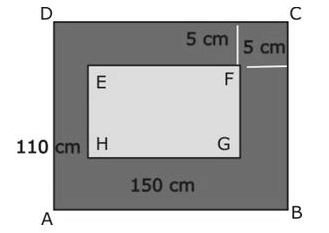Detailed Solution for Olympiad Test: Mensuration - Question 14

Area of Rectangle ABCD = 110*150 = 16500cm2
Area of rectangle EFGH = (150-10)(110-10) = 14000cm2
Area of path = 16500-14000 = 2500cm2

Olympiad Test: Mensuration - Question 15

The area of a rhombus is 28 cm2 and one of its diagonals is 4 cm. Find its perimeter.

Olympiad Test: Mensuration - Question 16

Find the side of a cube whose surface area is 2400 cm2.

Olympiad Test: Mensuration - Question 17

Find the height of a cuboid whose volume is 275 cm3 and base area is 25 cm2

Detailed Solution for Olympiad Test: Mensuration - Question 17

Area of the base = Product of length and breadth

Volume of cuboid = Length x breadth x Height
= Area of its base x Height
275 = 25 x Height
Height = 275 / 25 cm
=11 cm

Olympiad Test: Mensuration - Question 18

Find the height of cuboid whose volume is 490 cm3 and base area is 35 cm2

Detailed Solution for Olympiad Test: Mensuration - Question 18

Volume of cuboid = base area × height
490 = 35 × height
height = 14cm.

Olympiad Test: Mensuration - Question 19

Surface area of a cube = _________

Olympiad Test: Mensuration - Question 20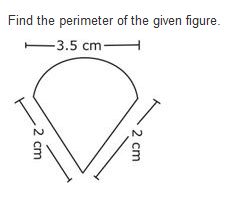Detailed Solution for Olympiad Test: Mensuration - Question 20

Circumference of semi circle =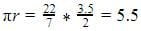perimeter = sum of two sides + circumference = 4 + 5.5 = 9.5cm

## Mathematics (Maths) Class 8

187 videos|321 docs|48 tests
 Use Code STAYHOME200 and get INR 200 additional OFF Use Coupon Code